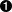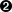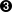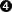## 3.2. Introducing Lists

Lists are Python's workhorse datatype. If your only experience with lists is arrays in Visual Basic or (God forbid) the datastore in Powerbuilder, brace yourself for Python lists.A list in Python is like an array in Perl. In Perl, variables that store arrays always start with the @ character; in Python, variables can be named anything, and Python keeps track of the datatype internally.A list in Python is much more than an array in Java (although it can be used as one if that's really all you want out of life). A better analogy would be to the ArrayList class, which can hold arbitrary objects and can expand dynamically as new items are added.

### Example 3.6. Defining a List

```>>> li = ["a", "b", "mpilgrim", "z", "example"]>>> li
['a', 'b', 'mpilgrim', 'z', 'example']
>>> li'a'
>>> li'example'```First, you define a list of five elements. Note that they retain their original order. This is not an accident. A list is an ordered set of elements enclosed in square brackets.A list can be used like a zero-based array. The first element of any non-empty list is always li.The last element of this five-element list is li, because lists are always zero-based.

### Example 3.7. Negative List Indices

```>>> li
['a', 'b', 'mpilgrim', 'z', 'example']
>>> li[-1]'example'
>>> li[-3]'mpilgrim'```A negative index accesses elements from the end of the list counting backwards. The last element of any non-empty list is always li[-1].If the negative index is confusing to you, think of it this way: li[-n] == li[len(li) - n]. So in this list, li[-3] == li[5 - 3] == li.

### Example 3.8. Slicing a List

```>>> li
['a', 'b', 'mpilgrim', 'z', 'example']
>>> li[1:3]['b', 'mpilgrim']
>>> li[1:-1]['b', 'mpilgrim', 'z']
>>> li[0:3]['a', 'b', 'mpilgrim']```You can get a subset of a list, called a “slice”, by specifying two indices. The return value is a new list containing all the elements of the list, in order, starting with the first slice index (in this case li), up to but not including the second slice index (in this case li).Slicing works if one or both of the slice indices is negative. If it helps, you can think of it this way: reading the list from left to right, the first slice index specifies the first element you want, and the second slice index specifies the first element you don't want. The return value is everything in between.Lists are zero-based, so li[0:3] returns the first three elements of the list, starting at li, up to but not including li.

### Example 3.9. Slicing Shorthand

```>>> li
['a', 'b', 'mpilgrim', 'z', 'example']
>>> li[:3]['a', 'b', 'mpilgrim']
>>> li[3:]['z', 'example']
>>> li[:]['a', 'b', 'mpilgrim', 'z', 'example']```If the left slice index is 0, you can leave it out, and 0 is implied. So li[:3] is the same as li[0:3] from Example 3.8, “Slicing a List”.Similarly, if the right slice index is the length of the list, you can leave it out. So li[3:] is the same as li[3:5], because this list has five elements.Note the symmetry here. In this five-element list, li[:3] returns the first 3 elements, and li[3:] returns the last two elements. In fact, li[:n] will always return the first n elements, and li[n:] will return the rest, regardless of the length of the list.If both slice indices are left out, all elements of the list are included. But this is not the same as the original li list; it is a new list that happens to have all the same elements. li[:] is shorthand for making a complete copy of a list.

### Example 3.10. Adding Elements to a List

```>>> li
['a', 'b', 'mpilgrim', 'z', 'example']
>>> li.append("new")>>> li
['a', 'b', 'mpilgrim', 'z', 'example', 'new']
>>> li.insert(2, "new")>>> li
['a', 'b', 'new', 'mpilgrim', 'z', 'example', 'new']
>>> li.extend(["two", "elements"])>>> li
['a', 'b', 'new', 'mpilgrim', 'z', 'example', 'new', 'two', 'elements']```append adds a single element to the end of the list.insert inserts a single element into a list. The numeric argument is the index of the first element that gets bumped out of position. Note that list elements do not need to be unique; there are now two separate elements with the value 'new', li and li.extend concatenates lists. Note that you do not call extend with multiple arguments; you call it with one argument, a list. In this case, that list has two elements.

### Example 3.11. The Difference between extend and append

```>>> li = ['a', 'b', 'c']
>>> li.extend(['d', 'e', 'f'])>>> li
['a', 'b', 'c', 'd', 'e', 'f']
>>> len(li)6
>>> li[-1]
'f'
>>> li = ['a', 'b', 'c']
>>> li.append(['d', 'e', 'f'])>>> li
['a', 'b', 'c', ['d', 'e', 'f']]
>>> len(li)4
>>> li[-1]
['d', 'e', 'f']
```Lists have two methods, extend and append, that look like they do the same thing, but are in fact completely different. extend takes a single argument, which is always a list, and adds each of the elements of that list to the original list.Here you started with a list of three elements ('a', 'b', and 'c'), and you extended the list with a list of another three elements ('d', 'e', and 'f'), so you now have a list of six elements.On the other hand, append takes one argument, which can be any data type, and simply adds it to the end of the list. Here, you're calling the append method with a single argument, which is a list of three elements.Now the original list, which started as a list of three elements, contains four elements. Why four? Because the last element that you just appended is itself a list. Lists can contain any type of data, including other lists. That may be what you want, or maybe not. Don't use append if you mean extend.

### Example 3.12. Searching a List

```>>> li
['a', 'b', 'new', 'mpilgrim', 'z', 'example', 'new', 'two', 'elements']
>>> li.index("example")5
>>> li.index("new")2
>>> li.index("c")Traceback (innermost last):
File "<interactive input>", line 1, in ?
ValueError: list.index(x): x not in list
>>> "c" in liFalse```index finds the first occurrence of a value in the list and returns the index.index finds the first occurrence of a value in the list. In this case, 'new' occurs twice in the list, in li and li, but index will return only the first index, 2.If the value is not found in the list, Python raises an exception. This is notably different from most languages, which will return some invalid index. While this may seem annoying, it is a good thing, because it means your program will crash at the source of the problem, rather than later on when you try to use the invalid index.To test whether a value is in the list, use in, which returns True if the value is found or False if it is not.Before version 2.2.1, Python had no separate boolean datatype. To compensate for this, Python accepted almost anything in a boolean context (like an if statement), according to the following rules: 0 is false; all other numbers are true. An empty string ("") is false, all other strings are true. An empty list ([]) is false; all other lists are true. An empty tuple (()) is false; all other tuples are true. An empty dictionary ({}) is false; all other dictionaries are true. These rules still apply in Python 2.2.1 and beyond, but now you can also use an actual boolean, which has a value of True or False. Note the capitalization; these values, like everything else in Python, are case-sensitive.

### Example 3.13. Removing Elements from a List

```>>> li
['a', 'b', 'new', 'mpilgrim', 'z', 'example', 'new', 'two', 'elements']
>>> li.remove("z")>>> li
['a', 'b', 'new', 'mpilgrim', 'example', 'new', 'two', 'elements']
>>> li.remove("new")>>> li
['a', 'b', 'mpilgrim', 'example', 'new', 'two', 'elements']
>>> li.remove("c")Traceback (innermost last):
File "<interactive input>", line 1, in ?
ValueError: list.remove(x): x not in list
>>> li.pop()'elements'
>>> li
['a', 'b', 'mpilgrim', 'example', 'new', 'two']```remove removes the first occurrence of a value from a list.remove removes only the first occurrence of a value. In this case, 'new' appeared twice in the list, but li.remove("new") removed only the first occurrence.If the value is not found in the list, Python raises an exception. This mirrors the behavior of the index method.pop is an interesting beast. It does two things: it removes the last element of the list, and it returns the value that it removed. Note that this is different from li[-1], which returns a value but does not change the list, and different from li.remove(value), which changes the list but does not return a value.

### Example 3.14. List Operators

```>>> li = ['a', 'b', 'mpilgrim']
>>> li = li + ['example', 'new']>>> li
['a', 'b', 'mpilgrim', 'example', 'new']
>>> li += ['two']>>> li
['a', 'b', 'mpilgrim', 'example', 'new', 'two']
>>> li = [1, 2] * 3>>> li
[1, 2, 1, 2, 1, 2]```Lists can also be concatenated with the + operator. list = list + otherlist has the same result as list.extend(otherlist). But the + operator returns a new (concatenated) list as a value, whereas extend only alters an existing list. This means that extend is faster, especially for large lists.Python supports the += operator. li += ['two'] is equivalent to li.extend(['two']). The += operator works for lists, strings, and integers, and it can be overloaded to work for user-defined classes as well. (More on classes in Chapter 5.)The * operator works on lists as a repeater. li = [1, 2] * 3 is equivalent to li = [1, 2] + [1, 2] + [1, 2], which concatenates the three lists into one.